×#### Thank you for registering.

One of our academic counsellors will contact you within 1 working day.

Click to Chat

1800-1023-196

+91-120-4616500

CART 0

• 0

MY CART (5)

Use Coupon: CART20 and get 20% off on all online Study Material

ITEM
DETAILS
MRP
DISCOUNT
FINAL PRICE
Total Price: Rs.

There are no items in this cart.
Continue Shopping• Complete JEE Main/Advanced Course and Test Series
• OFFERED PRICE: Rs. 15,900
• View Details

Chapter 23 Data Handling II (Central Values) Exercise – 23.1

Question: 1

Ashish studies for 4 hours, 5 hours and 3 hours on three consecutive days. How many hours does he study daily on an average?

Solution:

Average number of study hours = (4 + 5 + 3) ÷ 3

= 12 ÷ 3

= 4 hours

Thus, Ashish studies for 4 hours on an average.

Question: 2

A cricketer scores the following runs in 8 innings: 58, 76, 40, 35, 48, 45, 0, 100.

Find the mean score.

Solution:

We have:

The mean score = (58 + 76 + 40 + 35 + 48 + 45 + 0 + 100) ÷ 8

= 402 ÷ 8

= 50.25 runs.

Question: 3

The marks (out of 100) obtained by a group of students in science test are 85, 76, 90, 84, 39, 48, 56, 95, 81 and 75. Find the

(i) Highest and the lowest marks obtained by the students.

(ii) Range of marks obtained.

(iii) Mean marks obtained by the group.

Solution:

In order to find the highest and lowest marks, let us arrange the marks in ascending order as follows:

39, 48, 56, 75, 76, 81, 84, 85, 90, 95

(i) Clearly, the highest mark is 95 and the lowest is 39.

(ii) The range of the marks obtained is: (95 – 39) = 56.

(iii) We have:

Mean marks = Sum of the marks ÷ Total number of students

→ Mean marks = (39 + 48 + 56 + 75 + 76 + 81 + 84 + 85 + 90 + 95) ÷ 10

= 729 ÷ 10

= 72.9.

Hence, the mean mark of the students is 72.9.

Question: 4

The enrolment of a school during six consecutive years was as follows:

1555, 1670, 1750, 2019, 2540, 2820

Find the mean enrollment of the school for this period.

Solution:

The mean enrolment = Sum of the enrolments in each year ÷ Total number of years

The mean enrolment = (1555 + 1670 + 1750 + 2019 + 2540 + 2820) ÷ 6

= 12354 ÷ 6

= 2059.

Thus, the mean enrolment of the school for the given period is 2059.

Question: 5

The rainfall (in mm) in a city on 7 days of a certain week was recorded as follows:

Day
Mon
Tue
Wed
Thu
Fri
Sat
Sun

Rainfall (in mm)
0.0
12.2
2.1
0.0
20.5
5.3
1.0

(i) Find the range of the rainfall from the above data.

(ii) Find the mean rainfall for the week.

(iii) On how many days was the rainfall less than the mean rainfall.

Solution:

(i) The range of the rainfall = Maximum rainfall – Minimum rainfall

= 20.5 – 0.0

= 20.5 mm.

(ii) The mean rainfall = (0.0 + 12.2 + 2.1 + 0.0 + 20.5 + 5.3 + 1.0) ÷ 7

= 41.1 ÷ 7

= 5.87 mm.

(iii) Clearly, there are 5 days (Mon, Wed, Thu, Sat and Sun), when the rainfall was less than the mean, i.e., 5.87 mm.

Question: 6

If the heights of 5 persons are 140 cm, 150 cm, 152 cm, 158 cm and 161 cm respectively, find the mean height.

Solution:

The mean height = Sum of the heights ÷ Total number of persons

= (140 + 150 + 152 + 158 + 161) ÷ 5

= 761 ÷ 5

= 152.2 cm.

Question: 7

Find the mean of 994, 996, 998, 1002 and 1000.

Solution:

Mean = Sum of the observations ÷ Total number of observations

Mean = (994 + 996 + 998 + 1002 + 1000) ÷ 5

= 4990 ÷ 5

= 998.

Question: 8

Find the mean of first five natural numbers.

Solution:

The first five natural numbers are 1, 2, 3, 4 and 5.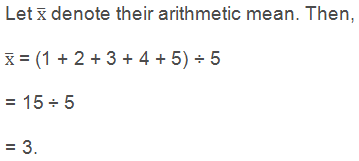Question: 9

Find the mean of all factors of 10.

Solution: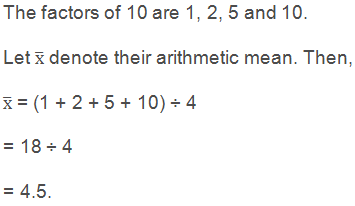Question: 10

Find the mean of first 10 even natural numbers.

Solution:Question: 11

Find the mean of x, x + 2, x + 4, x + 6, x + 8

Solution:

Mean = Sum of observations ÷ Number of observations

→ Mean = (x + x + 2 + x + 4 + x + 6 + x + 8) ÷ 5

→ Mean = (5x + 20) ÷ 5

→ Mean = 5(x + 4)5

→ Mean = x + 4

Question: 12

Find the mean of first five multiples of 3.

Solution:

The first five multiples of 3 are 3, 6, 9, 12 and 15.Question: 13

Following are the weights (in kg) of 10 new born babies in a hospital on a particular day: 3.4, 3.6, 4.2, 4.5, 3.9, 4.1, 3.8, 4.5, 4.4, 3.6 Find the mean

Solution:

We HaveQuestion: 14

The percentage of marks obtained by students of a class in mathematics are:

64, 36, 47, 23, 0, 19, 81, 93, 72, 35, 3, 1 Find their mean.

Solution:

We have.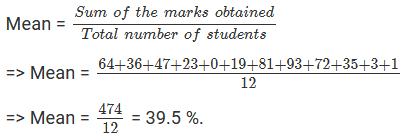Question: 15

The numbers of children in 10 families of a locality are:

2, 4, 3, 4, 2, 3, 5, 1, 1, 5 Find the mean number of children per family.

Solution: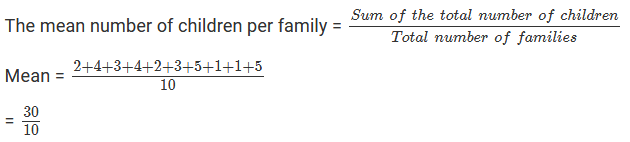= 3.

Thus, on an average there are 3 children per family in the locality.

Question: 16

The mean of marks scored by 100 students was found to be 40. Later on it was discovered that a score of 53 was misread as 83. Find the correct mean.

Solution:

We have:

n = The number of observations = 100, Mean = 40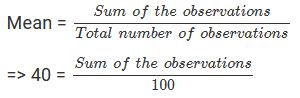→ Sum of the observations = 40 x 100

Thus, the incorrect sum of the observations = 40 x 100 = 4000.

Now,

The correct sum of the observations = Incorrect sum of the observations – Incorrect observation + Correct observation

→ The correct sum of the observations = 4000 – 83 + 53

→ The correct sum of the observations = 4000 – 30 = 3970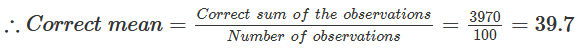Question: 17

The mean of five numbers is 27. If one number is excluded, their mean is 25. Find the excluded number.

Solution:

We have: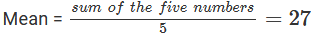So, sum of the five numbers = 5 x 27 = 135.

Now,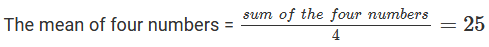So, sum of the four numbers = 4 x 25 = 100.

Therefore, the excluded number = Sum of the five number – Sum of the four numbers

→ The excluded number = 135 – 100 = 35.

Question: 18

The mean weight per student in a group of 7 students is 55 kg. The individual weights of 6 of them (in kg) are 52, 54, 55, 53, 56 and 54. Find the weight of the seventh student.

Solution:

We have: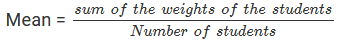Let the weight of the seventh student be x kg.Thus, the weight of the seventh student is 61 kg.

Question: 19

The mean weight of 8 numbers is 15 kg. If each number is multiplied by 2, what will be the new mean?

Solution:

Let x1, x2, x3…x8 be the eight numbers whose mean is 15 kg. Then,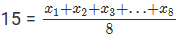x1 + x2 + x3 + …+ x8 = 15 × 8

→x1 + x2 + x3 +…+ x8 = 120.

Let the new numbers be 2x1, 2x2, 2x3 …2x8. Let M be the arithmetic mean of the new numbers.

Then,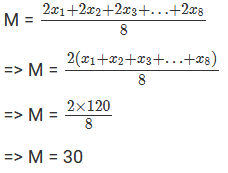Question: 20

The mean of 5 numbers is 18. If one number is excluded, their mean is 16. Find the excluded number.

Solution:

Let x1, x2, x3, x4 and x5 be five numbers whose mean is 18. Then,

18 = Sum of five numbers ÷ 5

∴ Sum of five numbers = 18 × 5 = 90

Now, if one number is excluded, then their mean is 16.

So,

16 = Sum of four numbers ÷ 4

∴ Sum of four numbers = 16 × 4 = 64.

The excluded number = Sum of five observations – Sum of four observations

∴ The excluded number = 90 – 64

∴ The excluded number = 26.

Question: 21

The mean of 200 items was 50. Later on, it was discovered that the two items were misread as 92 and 8 instead of 192 and 88. Find the correct mean.

Solution:

n = Number of observations = 200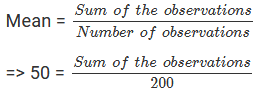→ Sum of the observations = 50 x 200 = 10,000.

Thus, the incorrect sum of the observations = 50 x 200

Now,

The correct sum of the observations = Incorrect sum of the observations – Incorrect observations + Correct observations

→ Correct sum of the observations = 10,000 – (92 + 8) + (192 + 88)

→ Correct sum of the observations = 10,000 – 100 + 280

→ Correct sum of the observations = 9900 + 280

→ Correct sum of the observations = 10,180.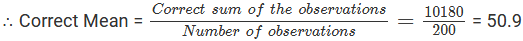Question: 22

The mean of 5 numbers is 27. If one more number is included, then the mean is 25. Find the included number.

Solution:

We have:

Mean = Sum of five numbers ÷ 5

→ Sum of the five numbers = 27 × 5 = 135.

Now, New mean = 25

25 = Sum of six numbers ÷ 6

→ Sum of the six numbers = 25 × 6 = 150.

The included number = Sum of the six numbers – Sum of the five numbers

→ The included number = 150 – 135

→ The included number = 15.

Question: 23

The mean of 75 numbers is 35. If each number is multiplied by 4, find the new mean.

Solution:

Let x1, x2, x3…x75 be 75 numbers with their mean equal to 35. Then,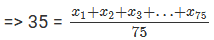x1 + x2 + x3 +…+x75 = 35 × 75

→ x1 + x2 + x3 +…+ x75 = 2625

The new numbers are 4 x 1, 4 x 2, 4 x 3…4 x 75

Let M be the arithmetic mean of the new numbers. Then,→ M = 140.### Course Features

• 728 Video Lectures
• Revision Notes
• Previous Year Papers
• Mind Map
• Study Planner
• NCERT Solutions
• Discussion Forum
• Test paper with Video Solution